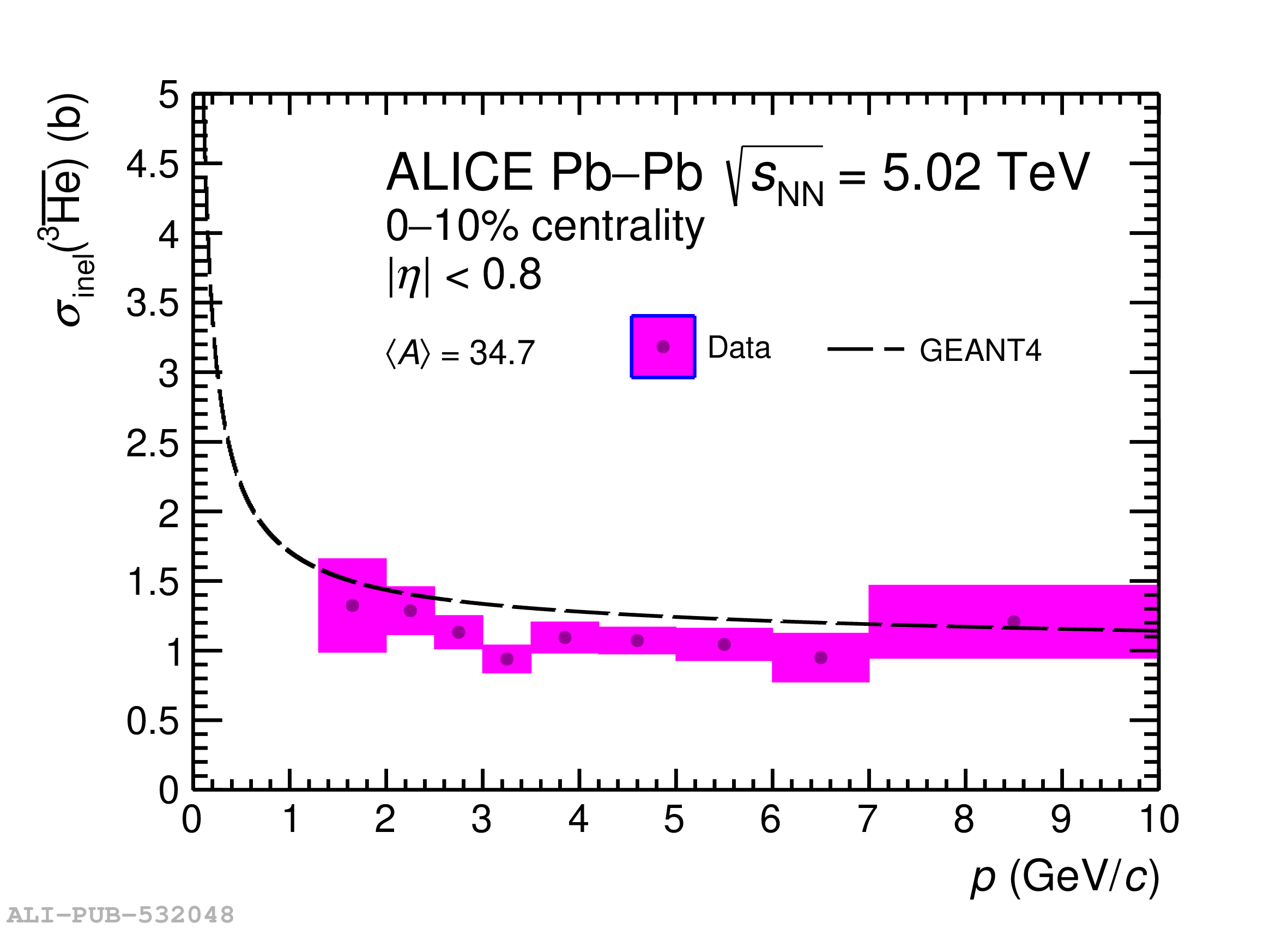# Figure 2

 Results for \sigmainel\ as a function of \ahe\ momentum. Results obtained from pp collisions at $\sqrt{s} =$ 13 TeV (left); results from the 10\% most central Pb--Pb collisions at $\sqrt{s_{\mathrm{NN}}} =\, 5.02$ TeV (right). The curves represent the \geant cross sections corresponding to the effective material probed by the different analyses. The arrow on the left plot shows the 95\% confidence limit on \sigmainel\ for $\langle A \rangle =$ 17.4. The different values of $\langle A \rangle$ correspond to the three different effective targets (see the main text for details). All the indicated uncertainties represent standard deviations.